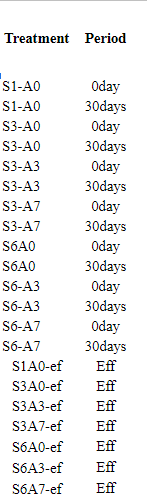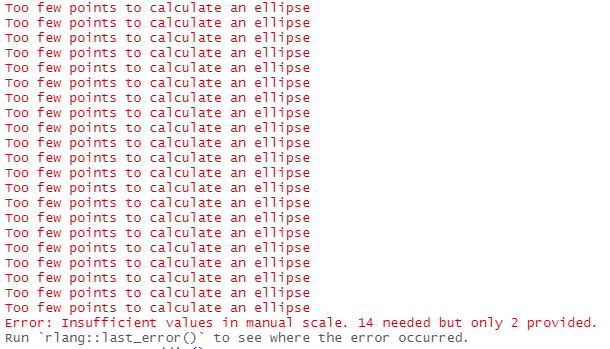# ggplot: coloring pcoa plot.

I made the pcoa plot through qiime2R, but the representation seem to all be same colors and my ellipsoid is just one surrounding the entire data. here is my code. please what should i fix here? @John_Blazier @Nicholas_Bokulich

uwunifrac\$data\$Vectors %>%

• left_join(shannon) %>%
• ggplot(aes(x=PC1, y=PC2, color= 'Period > 4', shape = 'Treatment', size= shannon_entropy)) + geom_point(alpha=0.5)+ xlab(paste(round(100uwunifrac\$data\$vectors,2),"%")) + ylab(paste("PC2: ", round(100uwunifrac\$data\$vectors), "%")) + theme_bw() + scale_shape_manual(values=c(17,1), name="Period") + scale_size_continuous(name="Shannon Diversity") + scale_color_discrete(name="Period") + ggtitle("Unweighted UniFrac") + stat_ellipse()

1 Like

Hi @pyghost,
Can you confirm that you actually have more than one level in both your `Period > r` column as well as the `shape` column? As it is, it can only recognize one level, which is why everything is in one color and one shape.

So example:

``````df <- uwunifrac\$data\$Vectors %>%
dplyr::left_join(shannon)

unique(df\$`Period > 4`)
unique(df\$Treatment)
``````

By the way, you can paste your R code here in a more readable way by sandwiching the code block by three backticks `````. So insert those quotes, enter for a new line, paste your code, enter again, and those 3 quotes again. Also you may want to check your code for pasting the x and y axis labels, they are currently not showing you what I think you want.

Here are my commands.

``````library(qiime2R)
library(tidyverse)
#> Rows: 21 Columns: 11
#> -- Column specification --------------------------------------------------------
#> Delimiter: "\t"
#> chr (3): SampleID, Treatment, Period
#> dbl (8): pH, Alkalinity_g CaCO3/kg, TAN(g N/kg), TVFAs_g/kg, TS, VS, daily_m...
#>
#> i Use `spec()` to retrieve the full column specification for this data.
#> i Specify the column types or set `show_col_types = FALSE` to quiet this message.
uwunifrac\$data\$Vectors %>%
+ left_join(shannon) %>%
+ ggplot(aes(x=PC1, y=PC2, color= 'Period > 4', shape = 'Treatment', size= shannon_entropy)) + geom_point(alpha=0.5)+ xlab(paste(round(100*uwunifrac\$data\$vectors,2),"%")) + ylab(paste("PC2: ", round(100*uwunifrac\$data\$vectors), "%")) + theme_bw() + scale_shape_manual(values=c(17,1), name="Period") + scale_size_continuous(name="Shannon Diversity") + scale_color_discrete(name="Period") + ggtitle("Unweighted UniFrac") + stat_ellipse()
#> Error in auto_copy(x, y, copy = copy): argument "y" is missing, with no default
``````

Created on 2021-11-23 by the reprex package (v2.0.1)

The command you suggested gave this

the columns for Period and Treatment has this.

Hi @pyghost,

There were quite a few little errors in the code so I made an example plot using Moving Pictures tutorial, see the code below and just change to your variable names where I made comments. Let me know how it goes!

``````library(qiime2R)
library(tidyverse)

#change filepaths if needed

df_join <- uwunifrac\$Vectors %>%
dplyr::select(SampleID, PC1, PC2) %>%
dplyr::left_join(shannon, by="SampleID")

df_join %>%
ggplot(aes(x=PC1, y=PC2,
color= `body-site`, #replace with Period
shape = `reported-antibiotic-usage`, #replace with Treatment
size= shannon_entropy)) +
geom_point(alpha=0.5) +
xlab(paste("PC1 (", round(100*uwunifrac\$ProportionExplained,2),"% variance explained)")) + #changed
ylab(paste("PC2 (", round(100*uwunifrac\$ProportionExplained,2),"% variance explained)")) + #changed
theme_bw() +
scale_shape_manual(values=c(17, 1), name="Antibiotic Useage") + #change to "Period"
scale_size_continuous(name="Shannon Diversity") + #keep as is
scale_color_discrete(name="Body Site") + #change to "Treatment"
ggtitle("Unweighted UniFrac")  +
stat_ellipse(inherit.aes = F, #in your example should be able to just use stat_ellipse()
aes(color=`body-site`, x=`PC1`, y=`PC2`))
``````

2 Likes

Hello @Mehrbod_Estaki, thank you so much for the support.
i ran the codes you sent with my parameters. it seem to run well but had this one error. i tried to reproduce it, but reprex kept crashing so i made screenshots.

and here was the error.
when i ran the code at this point, this was the result of the plot. the colors differences showed.

1 Like

Hi @pyghost,
The error is regarding the ellipses. See here for a mini discussion on this. I had the same issue with my demo which is why I decided to create the custom ellipses for demonstration purposes. My recommendation is to just not use ellipses since it doesn't make a whole lot of since with 2-4 points anyways1 Like

Thank you so much @Mehrbod_Estaki, i tried several means to fix that but didn't work. i will just go with your suggestion. Thanks again for your time and support.

1 Like